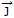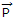# Electronics and Communication Engineering - Electromagnetic Field Theory - Discussion

Discussion Forum : Electromagnetic Field Theory - Section 10 (Q.No. 2)
2.
For EM wave propagation in free space, the free space is defined as
σ = 0, ε = 1, μ ≠ 1,= 0
σ = 0, ε = 1, μ = 1,= 0,= 0
σ ≠ 0, ε > 1, μ < 1,≠ 0,= 0
σ = 0, ε = 1, μ = 1,≠ 0,≠ 0